# Selina Solutions Concise Mathematics Class 6 Chapter 27: Quadrilateral Exercise 27(B)

Selina Solutions Concise Mathematics Class 6 Chapter 27 Quadrilateral Exercise 27(B) are formulated by the experts at BYJU’S to boost confidence among students. The solutions provide 100% accurate answers for each and every question included in this exercise. The main motto of creating solutions in an interactive manner is to make learning fun for students. By following these solutions, students undoubtedly secure more marks in the annual examination. To build a strong hold on these concepts, students can refer to Selina Solutions Concise Mathematics Class 6 Chapter 27 Quadrilateral Exercise 27(B) PDF free, from the links which are available below.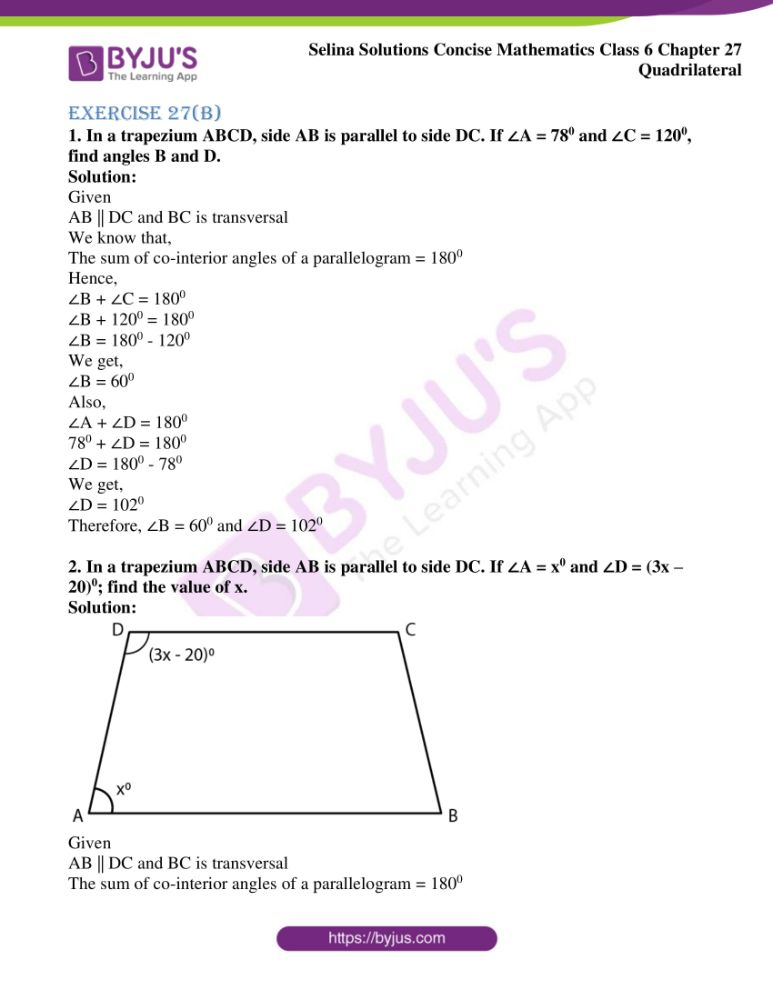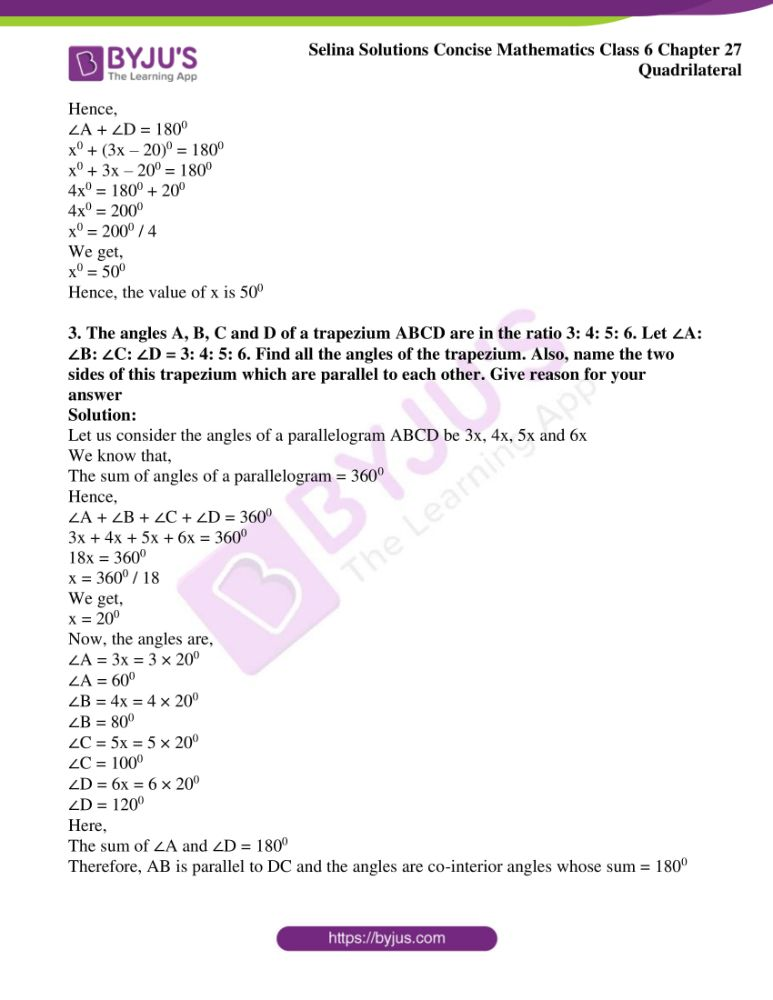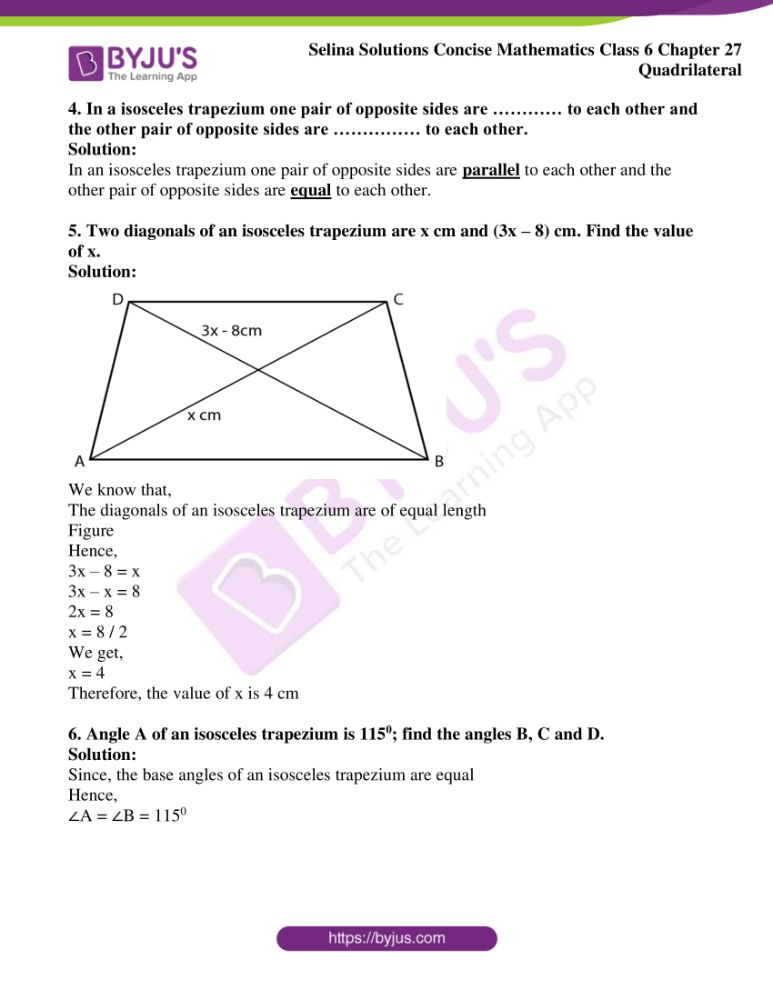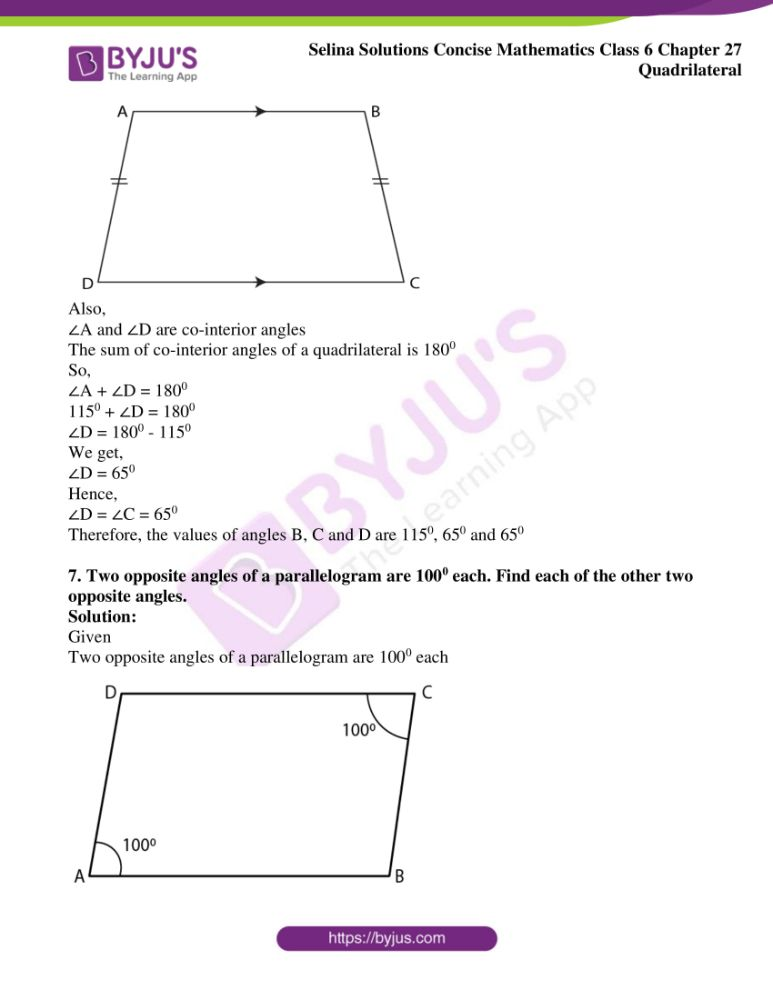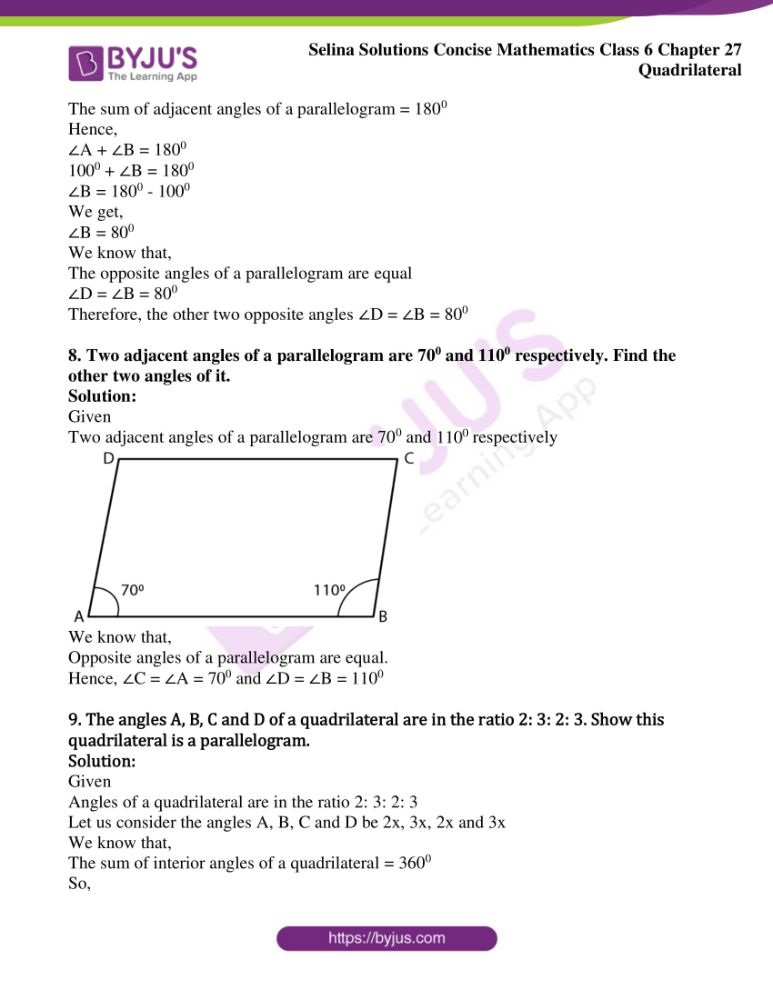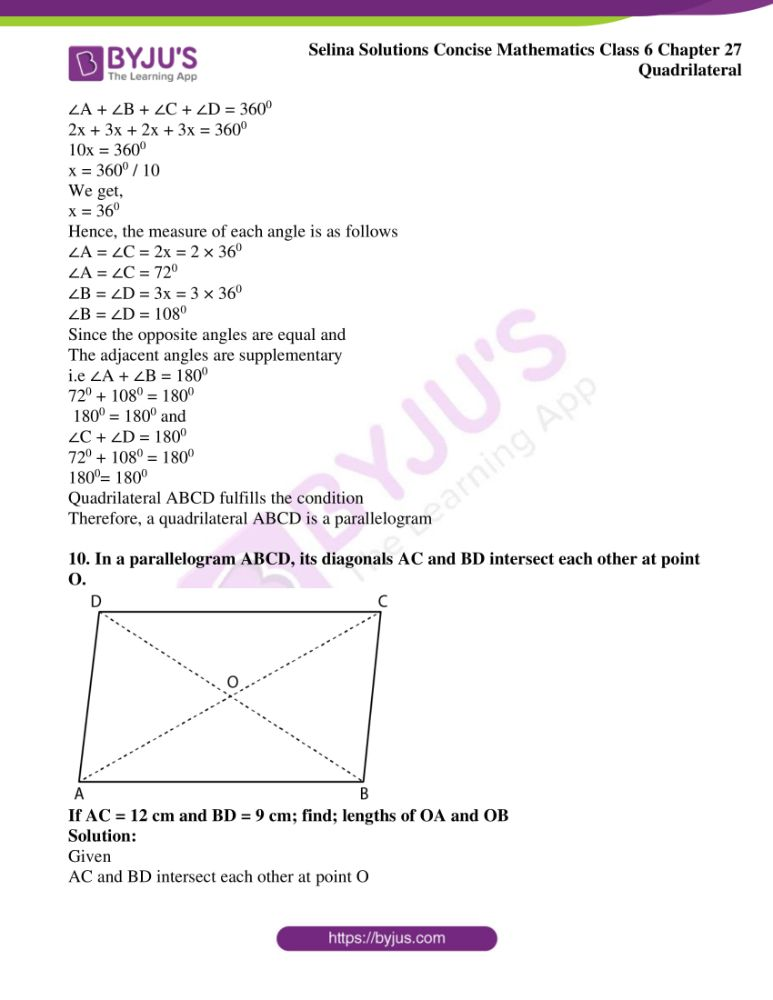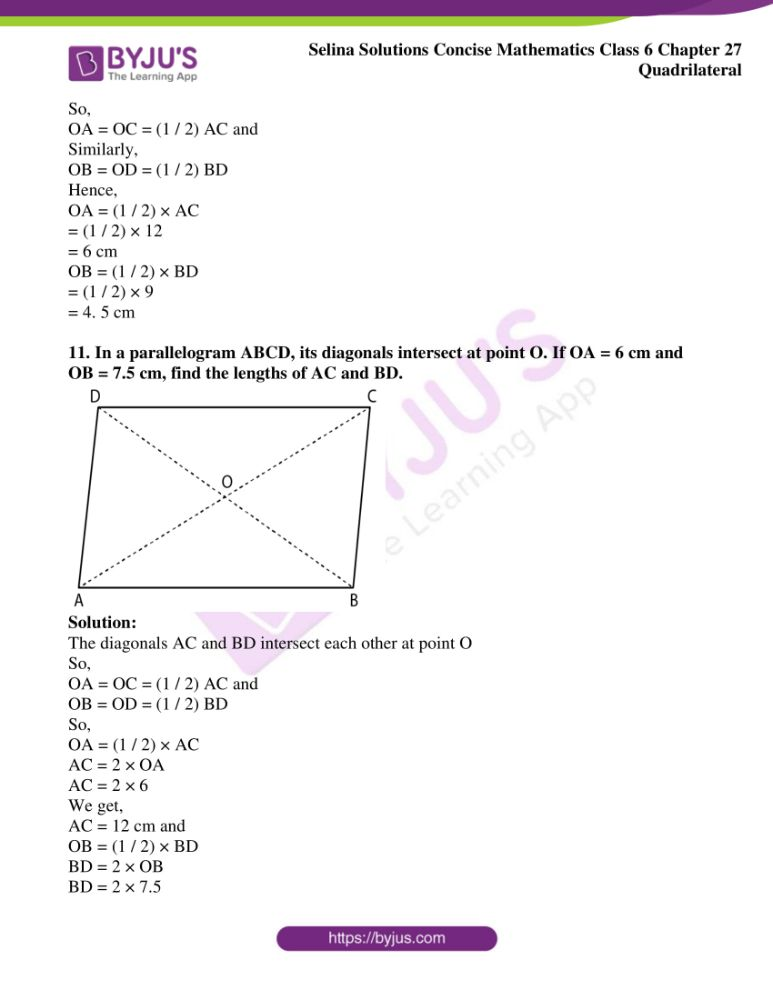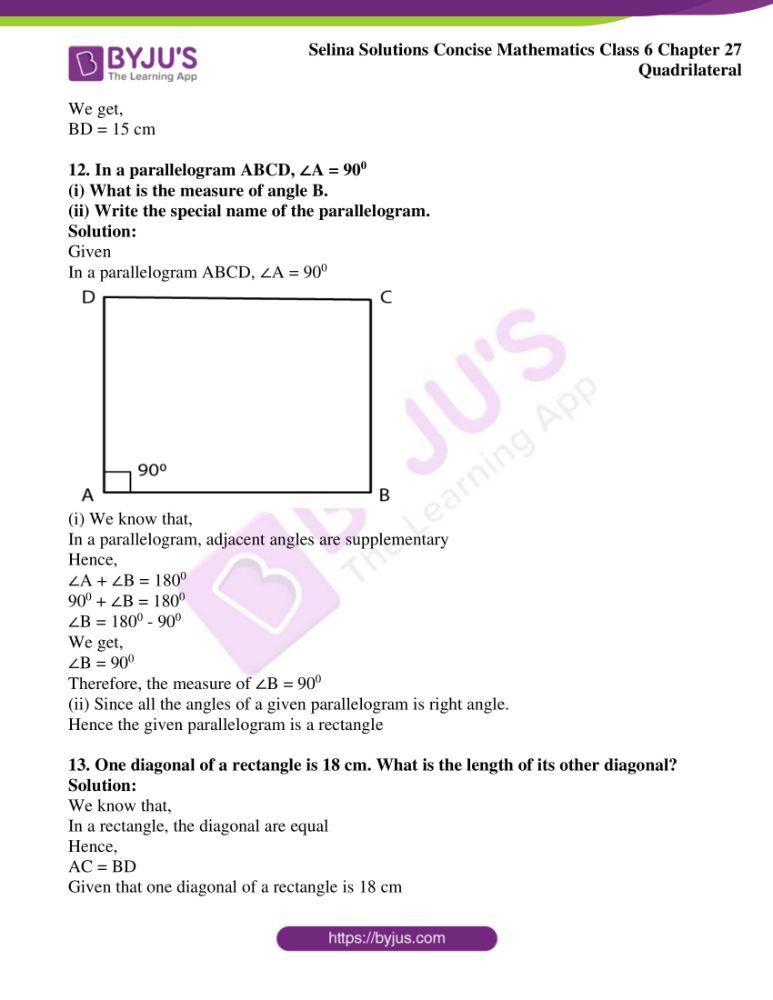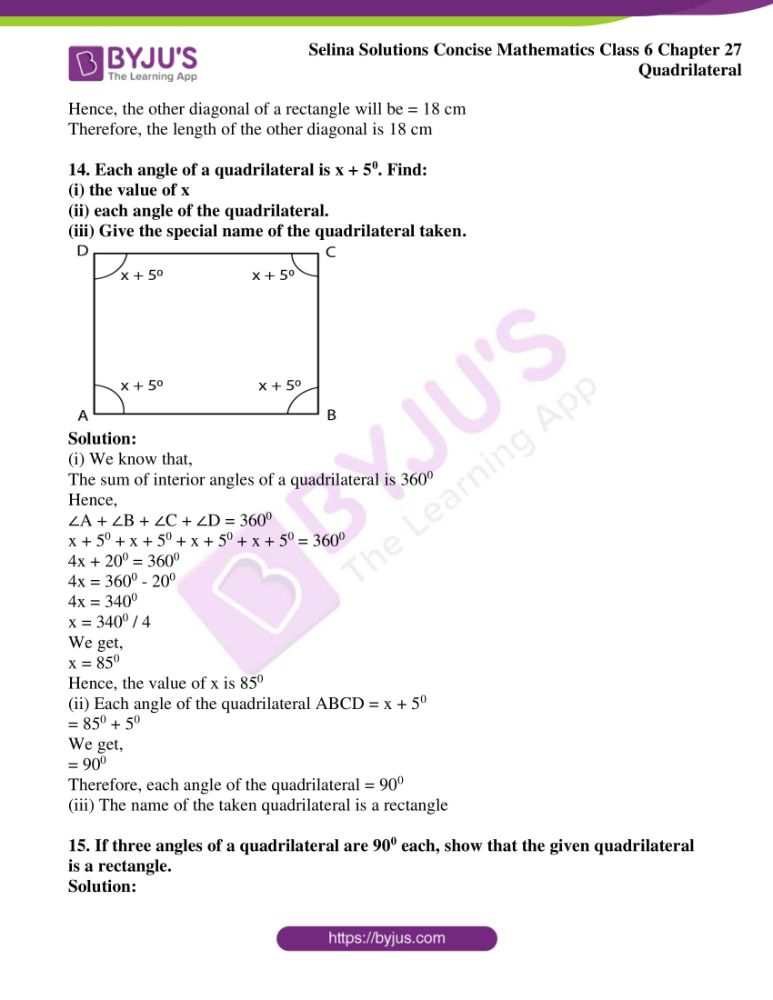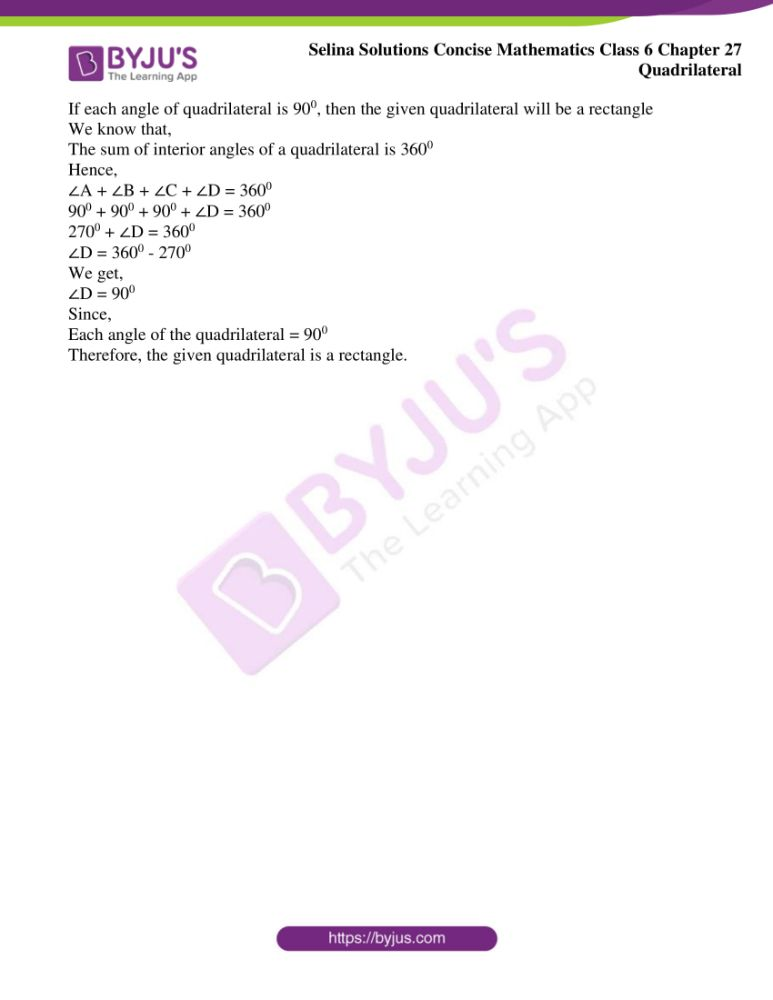### Access another exercise of Selina Solutions Concise Mathematics Class 6 Chapter 27: Quadrilateral

Exercise 27(A) Solutions

### Access Selina Solutions Concise Mathematics Class 6 Chapter 27 Quadrilateral Exercise 27(B)

Exercise 27(B)

1. In a trapezium ABCD, side AB is parallel to side DC. If ∠A = 780 and ∠C = 1200, find angles B and D.

Solution:

Given

AB || DC and BC is transversal

We know that,

The sum of co-interior angles of a parallelogram = 1800

Hence,

∠B + ∠C = 1800

∠B + 1200 = 1800

∠B = 1800 – 1200

We get,

∠B = 600

Also,

∠A + ∠D = 1800

780 + ∠D = 1800

∠D = 1800 – 780

We get,

∠D = 1020

Therefore, ∠B = 600 and ∠D = 1020

2. In a trapezium ABCD, side AB is parallel to side DC. If ∠A = x0 and ∠D = (3x – 20)0; find the value of x.

Solution: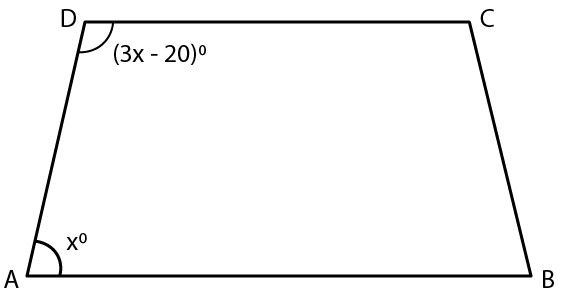Given

AB || DC and BC is transversal

The sum of co-interior angles of a parallelogram = 1800

Hence,

∠A + ∠D = 1800

x0 + (3x – 20)0 = 1800

x0 + 3x – 200 = 1800

4x0 = 1800 + 200

4x0 = 2000

x0 = 2000 / 4

We get,

x0 = 500

Hence, the value of x is 500

3. The angles A, B, C and D of a trapezium ABCD are in the ratio 3: 4: 5: 6. Let ∠A: ∠B: ∠C: ∠D = 3: 4: 5: 6. Find all the angles of the trapezium. Also, name the two sides of this trapezium which are parallel to each other. Give reason for your answer

Solution:

Let us consider the angles of a parallelogram ABCD be 3x, 4x, 5x and 6x

We know that,

The sum of angles of a parallelogram = 3600

Hence,

∠A + ∠B + ∠C + ∠D = 3600

3x + 4x + 5x + 6x = 3600

18x = 3600

x = 3600 / 18

We get,

x = 200

Now, the angles are,

∠A = 3x = 3 × 200

∠A = 600

∠B = 4x = 4 × 200

∠B = 800

∠C = 5x = 5 × 200

∠C = 1000

∠D = 6x = 6 × 200

∠D = 1200

Here,

The sum of ∠A and ∠D = 1800

Therefore, AB is parallel to DC and the angles are co-interior angles whose sum = 1800

4. In a isosceles trapezium one pair of opposite sides are ………… to each other and the other pair of opposite sides are …………… to each other.

Solution:

In an isosceles trapezium one pair of opposite sides are parallel to each other and the other pair of opposite sides are equal to each other.

5. Two diagonals of an isosceles trapezium are x cm and (3x – 8) cm. Find the value of x.

Solution: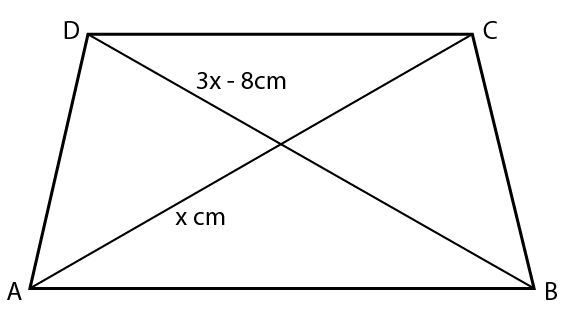We know that,

The diagonals of an isosceles trapezium are of equal length

Figure

Hence,

3x – 8 = x

3x – x = 8

2x = 8

x = 8 / 2

We get,

x = 4

Therefore, the value of x is 4 cm

6. Angle A of an isosceles trapezium is 1150; find the angles B, C and D.

Solution:

Since, the base angles of an isosceles trapezium are equal

Hence,

∠A = ∠B = 1150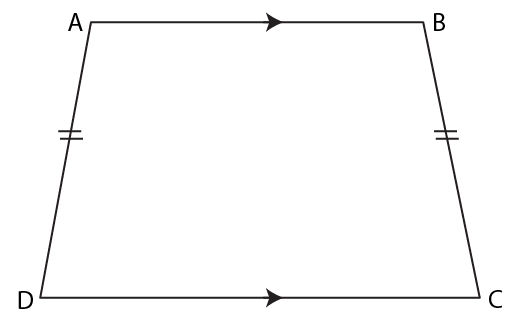Also,

∠A and ∠D are co-interior angles

The sum of co-interior angles of a quadrilateral is 1800

So,

∠A + ∠D = 1800

1150 + ∠D = 1800

∠D = 1800 – 1150

We get,

∠D = 650

Hence,

∠D = ∠C = 650

Therefore, the values of angles B, C and D are 1150, 650 and 650

7. Two opposite angles of a parallelogram are 1000 each. Find each of the other two opposite angles.

Solution:

Given

Two opposite angles of a parallelogram are 1000 each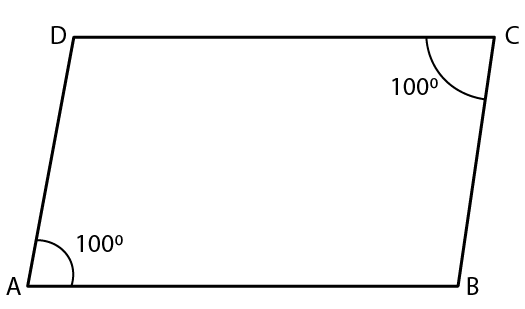The sum of adjacent angles of a parallelogram = 1800

Hence,

∠A + ∠B = 1800

1000 + ∠B = 1800

∠B = 1800 – 1000

We get,

∠B = 800

We know that,

The opposite angles of a parallelogram are equal

∠D = ∠B = 800

Therefore, the other two opposite angles ∠D = ∠B = 800

8. Two adjacent angles of a parallelogram are 700 and 1100 respectively. Find the other two angles of it.

Solution:

Given

Two adjacent angles of a parallelogram are 700 and 1100 respectively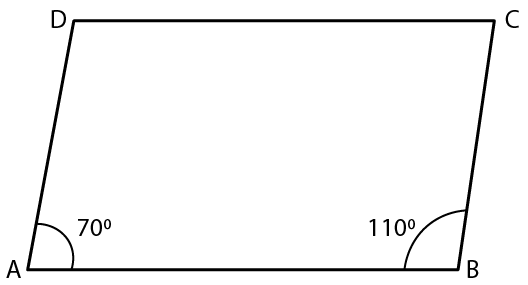We know that,

Opposite angles of a parallelogram are equal.

Hence, ∠C = ∠A = 700 and ∠D = ∠B = 1100

9. The angles A, B, C and D of a quadrilateral are in the ratio 2: 3: 2: 3. Show this quadrilateral is a parallelogram.

Solution:

Given

Angles of a quadrilateral are in the ratio 2: 3: 2: 3

Let us consider the angles A, B, C and D be 2x, 3x, 2x and 3x

We know that,

The sum of interior angles of a quadrilateral = 3600

So,

∠A + ∠B + ∠C + ∠D = 3600

2x + 3x + 2x + 3x = 3600

10x = 3600

x = 3600 / 10

We get,

x = 360

Hence, the measure of each angle is as follows

∠A = ∠C = 2x = 2 × 360

∠A = ∠C = 720

∠B = ∠D = 3x = 3 × 360

∠B = ∠D = 1080

Since the opposite angles are equal and

i.e ∠A + ∠B = 1800

720 + 1080 = 1800

1800 = 1800 and

∠C + ∠D = 1800

720 + 1080 = 1800

1800= 1800

Therefore, a quadrilateral ABCD is a parallelogram

10. In a parallelogram ABCD, its diagonals AC and BD intersect each other at point O.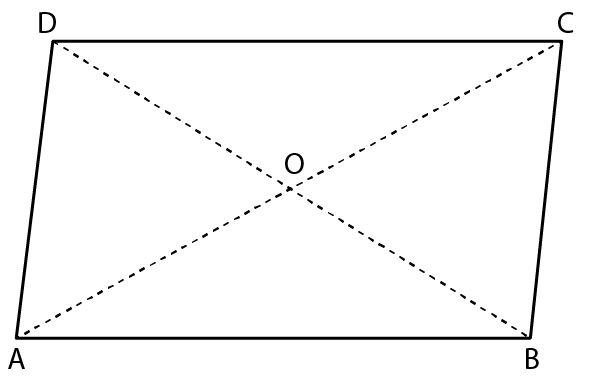If AC = 12 cm and BD = 9 cm; find; lengths of OA and OB

Solution:

Given

AC and BD intersect each other at point O

So,

OA = OC = (1 / 2) AC and

Similarly,

OB = OD = (1 / 2) BD

Hence,

OA = (1 / 2) × AC

= (1 / 2) × 12

= 6 cm

OB = (1 / 2) × BD

= (1 / 2) × 9

= 4. 5 cm

11. In a parallelogram ABCD, its diagonals intersect at point O. If OA = 6 cm and OB = 7.5 cm, find the lengths of AC and BD.Solution:

The diagonals AC and BD intersect each other at point O

So,

OA = OC = (1 / 2) AC and

OB = OD = (1 / 2) BD

So,

OA = (1 / 2) × AC

AC = 2 × OA

AC = 2 × 6

We get,

AC = 12 cm and

OB = (1 / 2) × BD

BD = 2 × OB

BD = 2 × 7.5

We get,

BD = 15 cm

12. In a parallelogram ABCD, ∠A = 900

(i) What is the measure of angle B.

(ii) Write the special name of the parallelogram.

Solution:

Given

In a parallelogram ABCD, ∠A = 900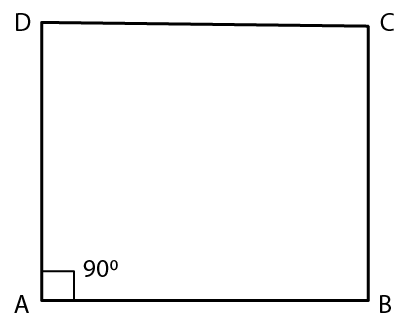(i) We know that,

In a parallelogram, adjacent angles are supplementary

Hence,

∠A + ∠B = 1800

900 + ∠B = 1800

∠B = 1800 – 900

We get,

∠B = 900

Therefore, the measure of ∠B = 900

(ii) Since all the angles of a given parallelogram is right angle.

Hence the given parallelogram is a rectangle

13. One diagonal of a rectangle is 18 cm. What is the length of its other diagonal?

Solution:

We know that,

In a rectangle, the diagonal are equal

Hence,

AC = BD

Given that one diagonal of a rectangle is 18 cm

Hence, the other diagonal of a rectangle will be = 18 cm

Therefore, the length of the other diagonal is 18 cm

14. Each angle of a quadrilateral is x + 50. Find:

(i) the value of x

(ii) each angle of the quadrilateral.

(iii) Give the special name of the quadrilateral taken.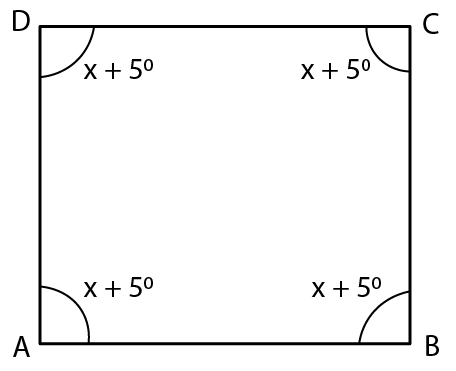Solution:

(i) We know that,

The sum of interior angles of a quadrilateral is 3600

Hence,

∠A + ∠B + ∠C + ∠D = 3600

x + 50 + x + 50 + x + 50 + x + 50 = 3600

4x + 200 = 3600

4x = 3600 – 200

4x = 3400

x = 3400 / 4

We get,

x = 850

Hence, the value of x is 850

(ii) Each angle of the quadrilateral ABCD = x + 50

= 850 + 50

We get,

= 900

Therefore, each angle of the quadrilateral = 900

(iii) The name of the taken quadrilateral is a rectangle

15. If three angles of a quadrilateral are 900 each, show that the given quadrilateral is a rectangle.

Solution:

If each angle of quadrilateral is 900, then the given quadrilateral will be a rectangle

We know that,

The sum of interior angles of a quadrilateral is 3600

Hence,

∠A + ∠B + ∠C + ∠D = 3600

900 + 900 + 900 + ∠D = 3600

2700 + ∠D = 3600

∠D = 3600 – 2700

We get,

∠D = 900

Since,

Each angle of the quadrilateral = 900

Therefore, the given quadrilateral is a rectangle.